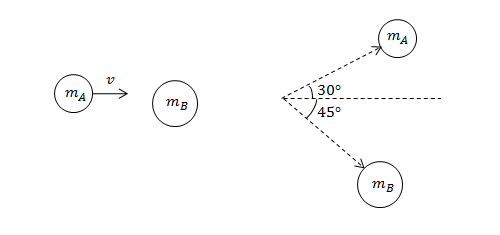Classical Mechanics

# Conservation of momentum

Two balls roll toward each other on a frictionless floor. The red ball has a mass of $1.00\text{ kg}$ and a speed of $3.00\text{ m/s}.$ The blue ball has a mass of $5.00\text{ kg}$ and a speed of $2.00 \text{ m/s}.$ If the balls stick together after the collision, determine the (approximate) final velocity of the balls.

A $200\text{ kg}$ bumper car travels at $8\text{ m/s}$ due east. It hits an identical bumper car traveling due north at $8\text{ m/s}.$ If the two cars lock bumpers and stick together, what is the approximate resulting speed of the cars?

An astronaut floating still in space has a total mass of $201\text{ kg},$ including his weight and all his equipment. He throws a $1\text{ kg}$ wrench with a speed of $10\text{ m/s}.$ What is the resulting speed of the astronaut?

Ball $A$ of mass $2\text{ kg}$ is rolling on a frictionless horizontal floor at $5\text{ m/s}.$ It collides head-on with ball $B$ of mass $3\text{ kg}$ which is initially at rest. If we let $v_A$ and $v_B$ denote the respective velocities of balls $A$ and $B$ after the collision, in which of the following situations are the linear momenta conserved?

Define the initial direction that ball $A$ moves in as positive direction.Object $A$ of mass $m_A=2\text{ kg}$ moves with a velocity of $v=2\text{ m/s}$ toward a stationary object $B$ of mass $m_B=4\text{ kg}.$ After the impact, objects $A$ and $B$ move off in directions that form $30^\circ$ and $45^\circ$ angles with the initial direction of object $A,$ respectively. Assuming that the system is entirely frictionless, what is the speed of object $A$ after the collision?

×